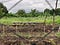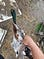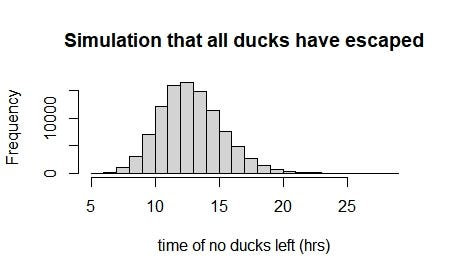Poisson Process: Four ducks vs. Anti-bird net

For the first time, I got interests in Poisson process based on a distribution “magnifique”: Poisson distribution. I was fascinated because of the process can fit in wide range of real life events, e.g. operation of consumer services, time of bus arrival, and the chance of a knight falling down from horseback. Today, I will introduce an interesting case happening in my real life: Four ducks vs. Anti-bird net.

Background

English version

Presently, I work in an organic French farm. The farmers decided to introduce Indian Runner Duck as a purpose of eating Slug in the field. To achieve that, the field needs to be surrounded by anti-bird net, so that the ducks would not escape from the field. However, due to the limit budget and workforce, farmers took a risk of duck escaping to just utilise the minimal materials for the surrounding, and see the efficiency of this system.

Version française

Maintenant, je travaille dans une ferme biologique. Les fermiers sont décidé d’introduire Canard coureur indien dans le but de manger limace dans le champ. Pour atteindre ce but, le champ a besoin d’être entouré par le filet, donc les canards ne s’échapperaient pas du champ. Pourtant, par suite du problème d’argent et la main d’oeuvre, les fermier sont pris un risque, et alors juste utilisé la structure simple pour le filet, et essayé l’efficacité de ce système.Figure 1: The ducks are finding food (slugs) in the field surrounded by the minimal anti-bird net.Figure 2: I am carrying a Indian Runner duck to the farm.

How to assess the efficiency of the anti-bird net?

To assess the efficiency of this system, I introduced Poisson process to the farmers (because I ate a great Poisson dish from them.) Using Poisson process, we can evaluate the system whether is efficiency, and have a potential to compare with the stronger anti-bird net will be utilised in the future.

At first, it is important to understand the expectation of duck escaping. For this data, I asked for the expectation from the farmers. “Combien de temps pense-toi qu’un canard trouvera un moyen de sortir? (English: How long do you think a duck will find a way out?)” I asked. Based on the rough estimate with a lot of emotional bias, they gave me a number: 10 hours for one duck. Secondly, I added up other data from the fact I knew, 4 ducks in this trial, and 16 hours in field per day (after that the farmers would push them into small chamber for sleeping). Finally, I get the required data for our evaluation.

After collecting the data, I need to define the question. It is explicit of estimating the expected time that all ducks escape from the anti-bird net, so that we can know after how long we will lose all of our ducks. Then, they work for a unit of three days, so they demand the situation while they check the number of ducks once in three days. Below is the precondition for this exciting calculation!

Information

1. Total number of ducks : 4
2. Expected escaping time per duck: 10 hours
3. The time period that ducks work in field: 16 hours per day

Question

1. What is the expected time that all ducks are all gone?
2. After 3-day work, how many ducks are expected still working for the farmers?

Thus, before we start the calculation, let me introduce Poisson distribution briefly.

Introduction of Poisson distribution

Poisson distribution is a discrete probability distribution, published by a French mathematician, Denis Poisson. It expresses the probability of a given number of events occurring in a fixed interval of time or space. The prerequisite is each event is independent and occurs with a known constant mean rate. The probability mass function (pmf) is expressed below:

where

• k is the number of occurrences (k=0,1,2…)
• e is Euler’s number
• ! is the factorial function.

Then, the most important characteristics of Poisson distribution is the constant mean rate λ is equivalent to expectation and variance of X.

Calculation

Expected time that all ducks have founded the way out

Firstly, the total ducks are four, and we (the farmers and I) interest in the time that the last duck is gone. Therefore, I randomly generate the hours that each duck has its possible time point of departure, and they follow poi(λ=10) with n =4. Then the return would be like T = (13,11,9,5). Subsequently, I select the maximal value as the result. At this point, one simulation has been done.

Following by that, I run hundreds of thousands of simulation to get the stable mean and variance. Here is some code from R below:

time_spend =c()
set.seed(123)
for (i in 1:100000) { # simulating for 100,000 times
duck_escape = rpois(4, 10) # 4 ducks sortent followed by poission distribution
all_duck_gone = max(duck_escape)
time_spend = c(time_spend ,all_duck_gone )
}
cat('mean:', mean(time_spend))
cat('varriance:',var(time_spend))
hist(time_spend, main= "Simulation of all ducks escaping", xlab = "time of no ducks left (hrs)")

Mean: 13.3313

Variance: 6.170152Figure 3: The histogram of the simulation that all ducks have escaped

After 3-day work, how many ducks are expected still working for the farmers?

In this question, it is necessary to look back to the pmf and slightly modify the equation. Instead of the average number of events λ, I rewrite it as the number of events r happened in a given time and the time length we would like to assign. Then the formula is like:

Afterwards, I start calculate the expected events that happened in our given time t. In this case, r is the reciprocal of λ, r = 1/λ = 1/10, and t is 3-day work, 3*16 = 48. However, the time points of departure for all ducks are independent, and for a duck, there are only 2 situation: departure (X = 1) or not departure (X=0). Following this train of thought, I calculate the expectation of departure for a duck. It means the possibility that it is still inside the net after 48 hours. Finally, adding up the expectation of four ducks, we would know the expected ducks are still inside the net and working for us.

The code below illustrates the process.

r = 1/10 #
t = 3*16
# k=0, a duck do not leave
no_leave = dpois(0, r*t)
# k=1 a duck leave
leave = 1-k0
duck_leave = no_leave*0 + leave*1# the total duck inside the net
4- duck_leave*4

After observing, hypothesizing, calculating the result is demonstrated in front of us. The net can keep ducks in the net for average 13.3313 hours, and after 3 working days, there is 0.03291899 in the net.

Afterwards story

C’est bon! je voudrais cocher si les canards travaillent bien!

After a hard-working brainstorming, I could not wait to tell the result to them, but before that, I went out to check the ducks if they were still in the farm. Here was the real situation in the field.

Merde! Il n’y a pas de canard dans le champ!

I yelled, ran, pushed aside the grass in order to find them. Finally, I found them in the back of the chamber, and herded them into the chamber.

“Bof! It is scaring! I was literally at the margin of being fired. They disappeared only 8 hours for the first time. C’est bizarre…" I thought.

To investigate on what happened. I start looking after them for couple of days after the event happened, and I found out something…

Mathematical simulation vs. Real life

As a matter of that, ducks are social animal. They stay in group! Therefore if there is one duck find the way out, it would want to get back, or it would attract other three to leave with it! There is not possible for a duck outside but other three inside the net…

D’accord! Voilà! Merci beaucoup! À demain!

Programmer | Agriculturist | Environmentalist